# If the oscilloscope in your experiment were measuring the potential difference across the resisto...

If the oscilloscope in your experiment were measuring the potential difference across the resistor instead of the capacitor and you plotted Vres/Vgen vs. log(frequency), what would you expect the plot to look like? Give a qualitative explanation for the behavior of this plot. Why is this set-up referred to as a “high pass filter”?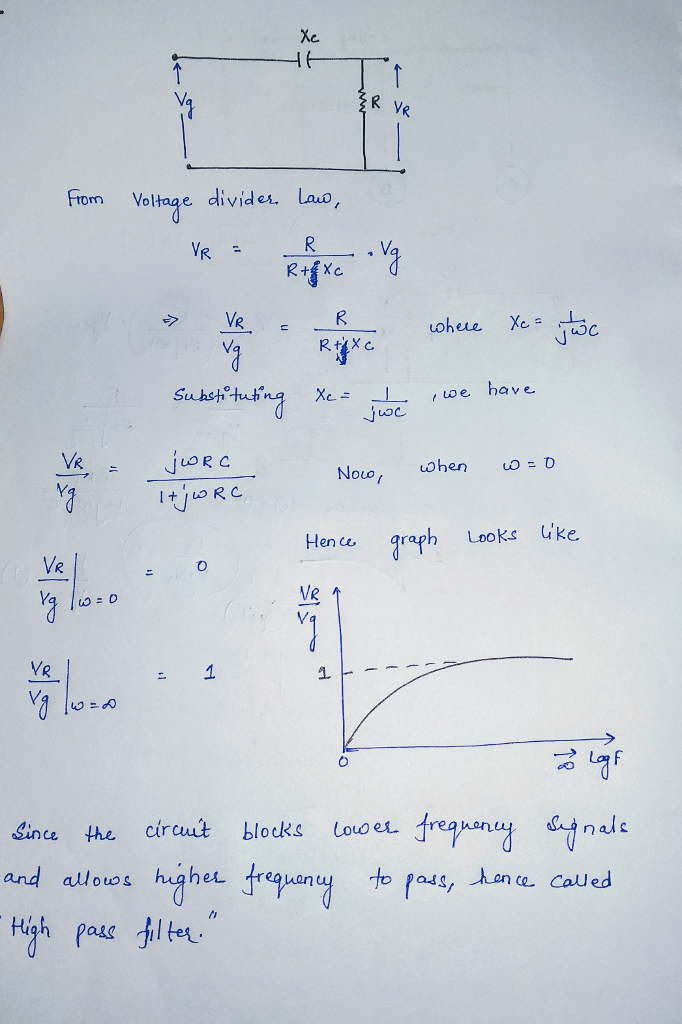##### Add Answer of: If the oscilloscope in your experiment were measuring the potential difference across the resisto...
Similar Homework Help Questions
• ### Plot of the natural logarithm of the potential across a capacitor vs. time?

How would the graph of a capacitor discharge look if you plotted the natural logarithm of the potential across the capacitor vs. time? Sketch a prediction.Also, what is the significance of the slope of the plot of ln(V) vs. time for a capacitor discharge circuit?( Keep in mind that the equation for capacitor discharge is V(t) = V0e-t/(RC) )

• ### EE 3342 Pre-Lab Exercises for Experiment 7: Passive Analog Filters Read the handout for Experimen...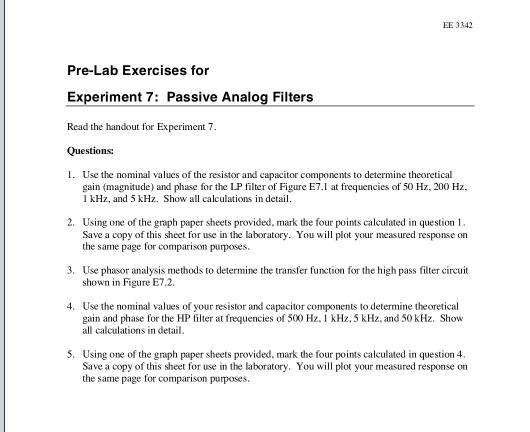E7.1 E7.2 EE 3342 Pre-Lab Exercises for Experiment 7: Passive Analog Filters Read the handout for Experiment 7. Questions: 1. Use the nominal values of the resistor and capacitor components to determine theoretical gain (magnitude) and phase for the LP filter of Figure E7.1 at frequencies of 50 Hz, 200 Hz, I kHz, and 5 kHz. Show all calculations in detail. 2. Using one of the graph paper sheets provided, mark the four points calculated in question Save a copy...

• ### 21 Vi Z2 Vo Figure 1 1. Ref: Figure 1. Let Z1 L (an inductor), Z2 - R (a resistor). Vi Calculate the magnitude and phase of the transfer function H(w) Figure 1 T 2. Repeat #1 with L = 100 mH, R 1kΩ....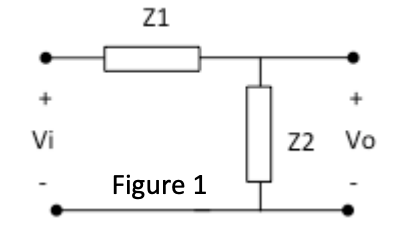21 Vi Z2 Vo Figure 1 1. Ref: Figure 1. Let Z1 L (an inductor), Z2 - R (a resistor). Vi Calculate the magnitude and phase of the transfer function H(w) Figure 1 T 2. Repeat #1 with L = 100 mH, R 1kΩ. a) Plot the frequency response in dB* on a both on a linear scale and then a log scale from ω-1 to 100,000,000 rad/sec with points every decade (1 b) 1,000 etc). 10 100 Plot the...

• ### Simulation For each filter mentioned in the following cases, first simulate the circuit using Multisim. You can get a plot of the transfer function that is called the Bode plot. From the right toolba...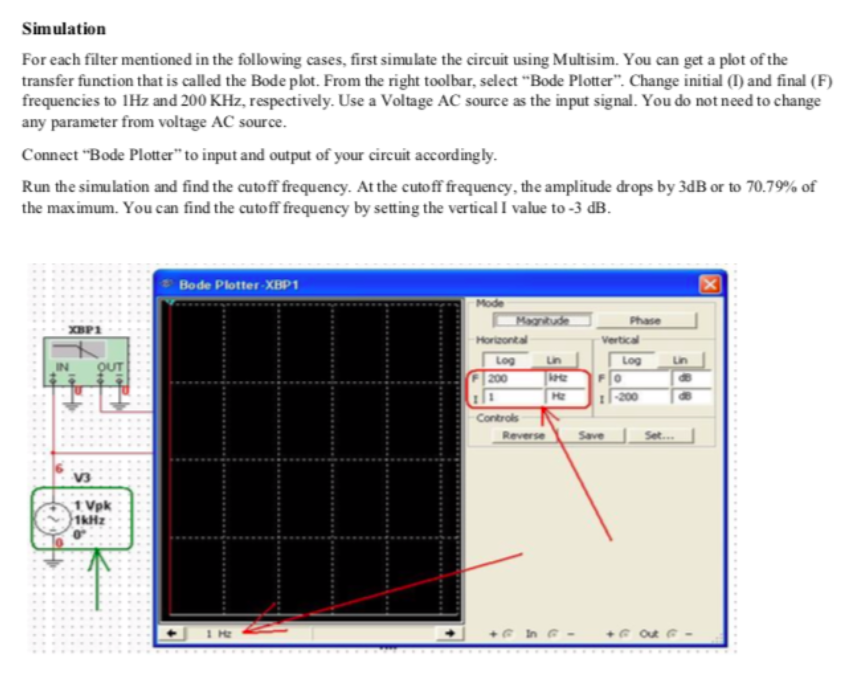Simulation For each filter mentioned in the following cases, first simulate the circuit using Multisim. You can get a plot of the transfer function that is called the Bode plot. From the right toolbar, select "Bode Plotter". Change initial (I) and final (F) frequencies to 1Hz and 200 KHz, respectively. Use a Voltage AC source as the input signal. You do not need to change any parameter from voltage AC source. Connect "Bode Plotter" to input and output of your...

• ### For each filter mentioned in the following cases, first simulate the circuit using Multisim. You can get a plot of the transfer function that is called the Bode plot. From the right toolbar, select &...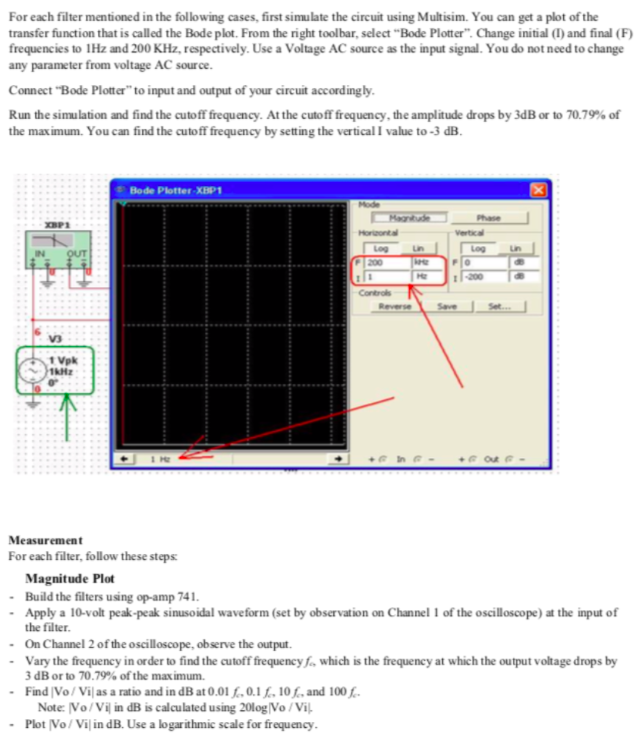For each filter mentioned in the following cases, first simulate the circuit using Multisim. You can get a plot of the transfer function that is called the Bode plot. From the right toolbar, select "Bode Plotter". Change initial (I) and final (F frequencies to 1Hz and 200 KHz, respectively. Use a Voltage AC source as the input signal. You do not need to change any parameter from voltage AC source Connect "Bode Plotter" to input and output of your circuit...

• ### help needed Problem 5 (40 points): LRC resonance an oscilloscope is used to measure the voltage drop ion generator e resistor of an LRC series circuit driven at varying AC frequency using a funct...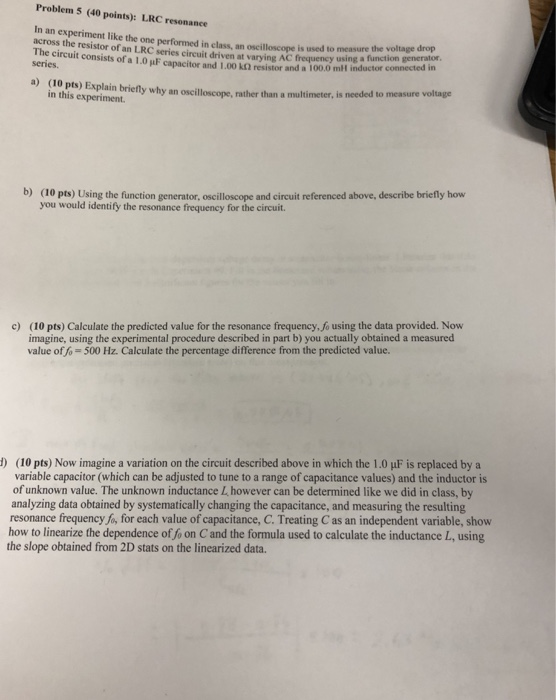help needed Problem 5 (40 points): LRC resonance an oscilloscope is used to measure the voltage drop ion generator e resistor of an LRC series circuit driven at varying AC frequency using a functi circuit consists of a 1.0 jiF capacitor and 1.00 k2 resistor and a 100.0 mll inductor c series. a) (10 pts) Explain briefty why an oscilloscope, in this experiment. rather than a multimeter, is needed to measure voltage (10 pts) Using the function generator, oscilloscope and...

• ### What are the Equilibrium voltages of the capacitor and the resistor when the function generator v...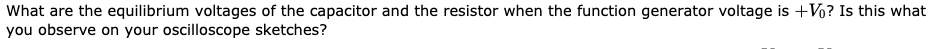What are the Equilibrium voltages of the capacitor and the resistor when the function generator voltage is +v0? Is this what you observe on your oscilloscope sketches? what are the equilibrium voltages of the capacitor and the resistor when the function generator voltage is ± you observe on your oscilloscope sketches? ? Is this what An RC circuit consists of a resistor connected to a capacitor. There may also be a fixed power supply, depending on whether the capacitor is...

• ### is it ok to use R1=4.7Kohms and C1=330pf? And I want to know how to calculate the R2, Rsrc, and C2 tage 2:Baseband Filt...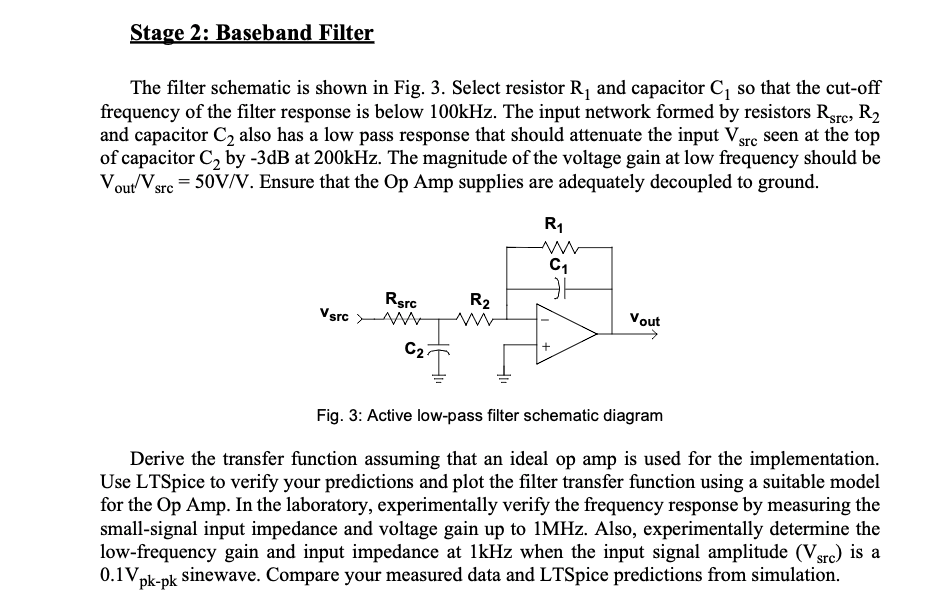is it ok to use R1=4.7Kohms and C1=330pf? And I want to know how to calculate the R2, Rsrc, and C2 tage 2:Baseband Filter The filter schematic is shown in Fig. 3. Select resistor Rj and capacitor C1 so that the cut-off frequency of the filter response is below 100kHz. The input network formed by resistors Rsrc, R2 and capacitor C2 also has a low pass response that should attenuate the input Vsrc seen at the top of capacitor C2...

• ### Plot or sketch the magnitude vs. frequency and the phase vs. frequency curves in a linear or log ...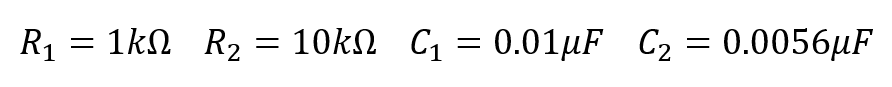Plot or sketch the magnitude vs. frequency and the phase vs. frequency curves in a linear or log scale. Indicate if the circuit is a low pass, high pass, band pass or band reject filter. We were unable to transcribe this image1 C1 R2 C2 Vout in 1 C1 R2 C2 Vout in

• ### Please answer using Matlab Software. Also please show the graphs in Matlab along with the Matlab ...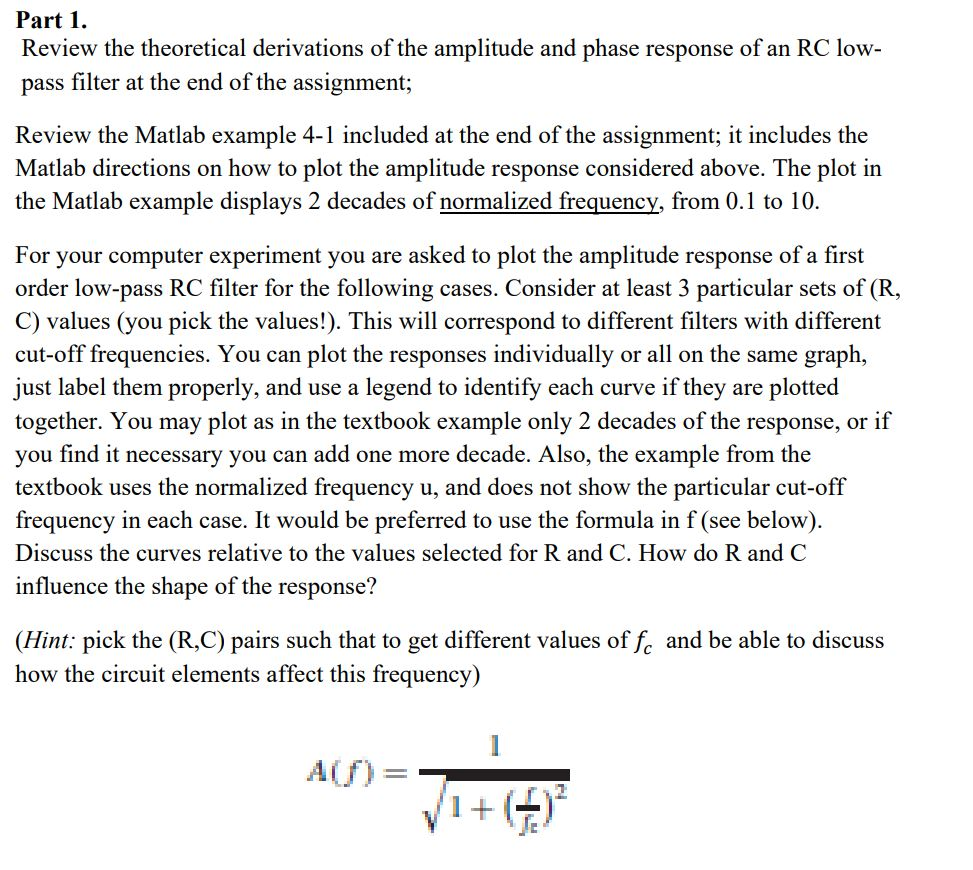Please answer using Matlab Software. Also please show the graphs in Matlab along with the Matlab Code. Thank you! Part 1 Review the theoretical derivations of the amplitude and phase response of an RC low pass filter at the end of the assignment; Review the Matlab example 4-1 included at the end of the assignment; it includes the Matlab directions on how to plot the amplitude response considered above. The plot in the Matlab example displays 2 decades of normalized...

Need Online Homework Help?